# Data partitions for boosting

The boosting algorithm can use two different forms of cross-validation: cross-validation as stopping criterion (always on), and cross-validation for model evaluation (optional). This requires paertitioning the data into different segments. The `eelbrain.plot.preview_partitions()` function is for exploring the effect of different parameters on the way the data is split.

## Validation

During boosting, every training step consists in modifying one element of the kernel/TRF. After every such step, the new TRF is evaluated against the validation data. For continuous data (without `Case` dimension), the default is to split the data into 10 equal-length segments, and perform 10 model fits, each using one of the segments as validation set. In the plots below, each “Split” shown on the y-axis corresponds to a separate run of the boosting algorithm. The results returned by the `boosting()` function would be based on to the average TRF of those 10 runs.

```# sphinx_gallery_thumbnail_number = 6
from eelbrain import *

p = plot.preview_partitions()
```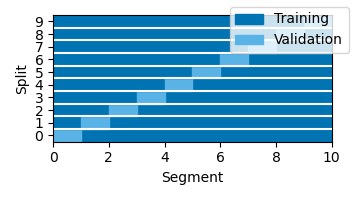The number of partitions can be controlled with the partitions parameter:

```p = plot.preview_partitions(partitions=5)
p = plot.preview_partitions(partitions=7)
```
•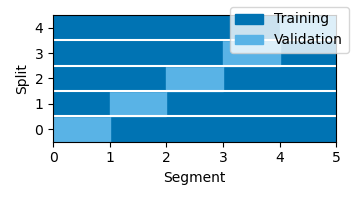•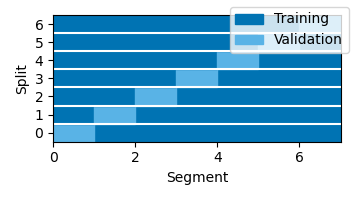For data with multiple trials (data with a `Case` dimension), the function attempts to use trials evenly across time:

```p = plot.preview_partitions(20, partitions=5)
p = plot.preview_partitions(20, partitions=2)
```
•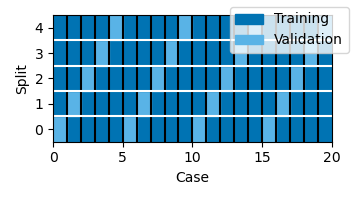•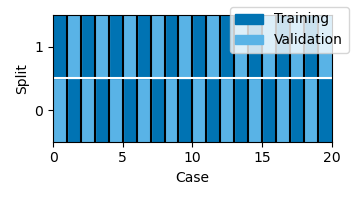## Testing

Testing the result of a model fit with cross-validation requires data that was never used during training. Testing with cross-validation is enabled in the `boosting()` function by setting `test=True`. When testing is enabled, each data segment is used in turn as testing segment. For each testing segment, the remaining segment are used in different runs as training and validation data. The results of those runs are then averaged to predict responses in the testing data. This nested loop means that the number of boosting runs can get large quickly when using many partitions, so the default is to use just four partitions:

```p = plot.preview_partitions(test=True)
p = plot.preview_partitions(20, partitions=5, test=True)
```
•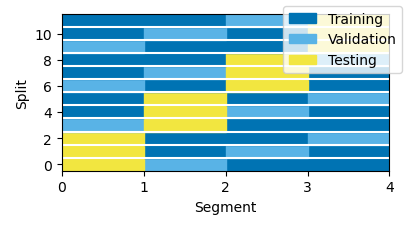•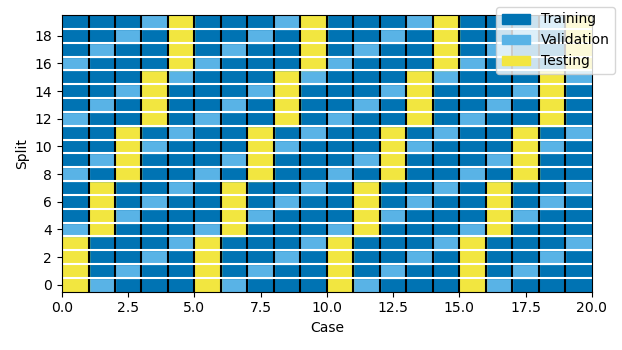Gallery generated by Sphinx-Gallery